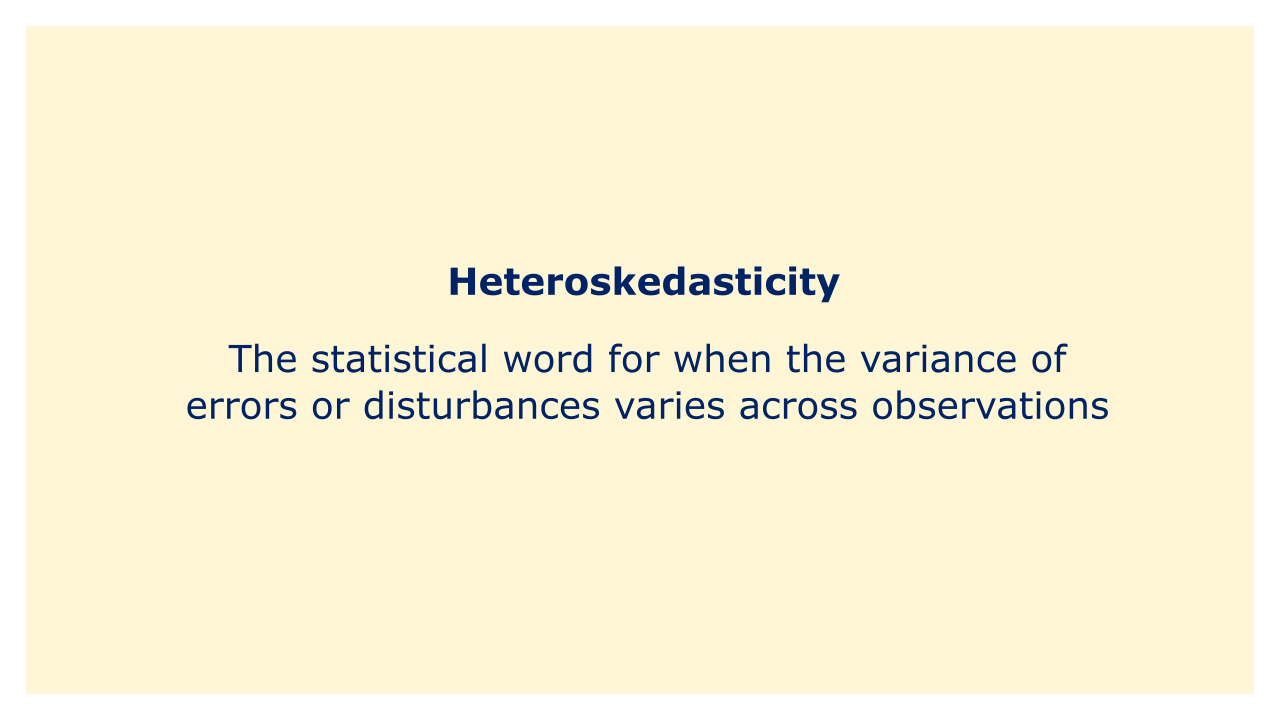# HeteroskedasticityImage: Moneybestpal.com

### In a regression model, heteroskedasticity is the statistical word for when the variance of errors or disturbances varies across observations. When a variable's variability rises or falls over the range of values of another variable, the assumption of homoscedasticity (equal variance) is violated.

To put it another way, heteroskedasticity is the uneven distribution of errors in a regression model where the dependent variable's variability varies over the range of the independent variable. Measurement errors, changes in data collection techniques, or modifications in the fundamental relationship between variables are only a few causes of this phenomenon.

Regression model estimate and interpretation may be significantly impacted by heteroskedasticity. It can, specifically, result in biased coefficient estimates and incorrect standard errors, which can lead to inaccurate hypothesis tests and confidence ranges. Consequently, while evaluating data, it is crucial to identify and compensate for heteroskedasticity.

Visual inspection of residual plots, statistical tests like the Breusch-Pagan test, utilizing robust standard errors, and other techniques can all be used to identify and correct heteroskedasticity. Reducing heteroskedasticity can enhance the precision and dependability of statistical models, resulting in more insightful conclusions and better decision-making.
Tags